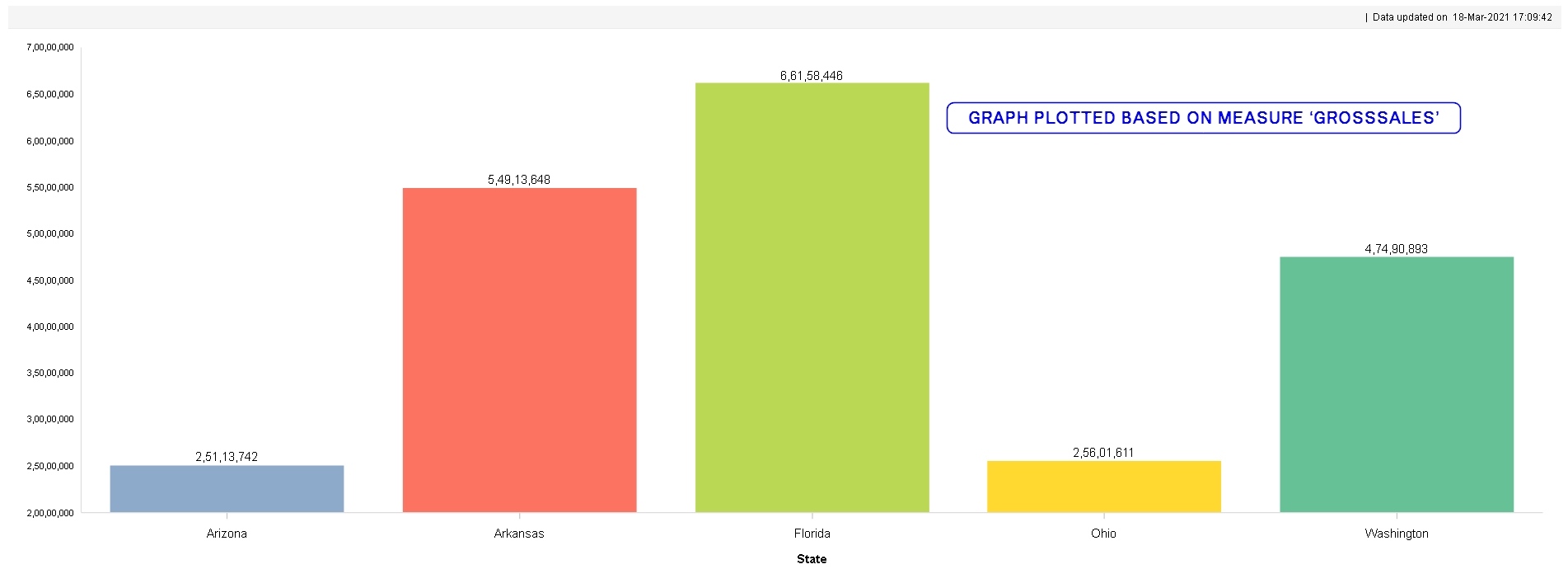1. Derive the total number of days within a selected date range by excluding months (as per the requirement)

Description:

Calculate the total number of days between two dates excluding April and May from the date range. For Ex; if the start date is 01-Feb-2020 and the end date is 01-Jun-2020, then the total number of days should be 59 days excluding Apr and May.

Example:

 Start Date End Date Result 01-Feb-2020 01-Jun-2020 59 days

UDDC Expression:

 ifCase(month(\$From_Date\$)>=6,noOfMonthsByDate(\$From_Date\$, \$To_Date\$),ifCase(month(\$To_Date\$)<=3,noOfMonthsByDate(\$From_Date\$, \$To_Date\$),ifCase((month(\$From_Date\$)>=1     && month(\$From_Date\$)<=4) &&   month(\$To_Date\$)==4 ,noOfMonthsByDate(\$From_Date\$, \$To_Date\$)-1,ifCase(month(\$From_Date\$)==4  &&      month(\$To_Date\$)==5 ,noOfMonthsByDate(\$From_Date\$, \$To_Date\$)-2,ifCase(month(\$From_Date\$)==5     &&   month(\$To_Date\$)==5 ,noOfMonthsByDate(\$From_Date\$, \$To_Date\$)-1,ifCase(month(\$From_Date\$)>=5     &&   month(\$To_Date\$)<=12 ,noOfMonthsByDate(\$From_Date\$, \$To_Date\$)-1,ifCase(month(\$From_Date\$)>=1 &&   (month(\$To_Date\$)>=5 && month(\$To_Date\$)<=12) ,noOfMonthsByDate(\$From_Date\$, \$To_Date\$)-2,0)))))    ))

Here, \$From_Date\$ and \$To_Date\$ are global variables through which the dates are selected.

2. Derive achievement % from target and actual values

Description:

Find out the achievement percentage from actual and target values to calculate the performance.

Example:

 Gross Sales Target Performance 70 100 70%

UDDC Expression:

Considering Sales example here;

 ifCase( (GrossSales == 0 ) && (Target != 0 ) , 0,                ifCase( (GrossSales != 0 ) && (Target == 0 ) , 100,                ifCase( (GrossSales == 0 ) && (Target == 0 ) , 0,((GrossSales * 100) / Target))))

3. Display measures in an object dynamically through global variables

Description:

Select measures dynamically and display in any object (Crosstab / Graph) from a list of measure values through global variables.

Example:

For example, in the below bar chart object, if you want to display different measures such as Gross Sales / Sales Qty / Discount based on the selection from Global variable, you can achieve them through the Global variable usage in the UDDC.GLOBAL VARIABLE LIST WITH MEASURE NAME VALUESGRAPH PLOTTED BASED ON GROSSSALES THAT IS SELECTED FROM THE LIST

As per the above figure, the user has chosen to view the ‘GrossSales’ from the global variables list, and the State wise Gross sales have been plotted in the graph.

User can select SalesQty to display State wise SalesQty in the same chart.

UDDC Expression:

A UDDC with the below expression has to be created and kept in the object data to achieve the same.

 ifCase( \$SelectMeasure\$ == "GrossSales", GrossSales,  ifCase( \$SelectMeasure\$ == "CostOfGoods", CostofGoods, SalesQty))